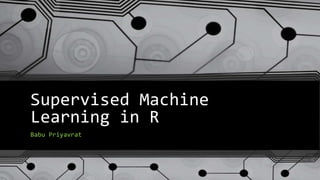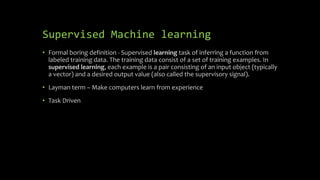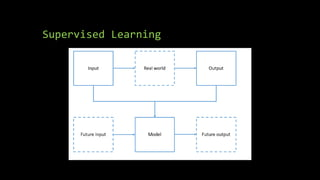Successfully reported this slideshow.

# Supervised Machine Learning in R×

# Supervised Machine Learning in R

Supervised Machine learning in R is discussed with R basics and how to clean, pre-process , partitioning. It also discusess some algorithms and how to control training itself using cross-validation.

Supervised Machine learning in R is discussed with R basics and how to clean, pre-process , partitioning. It also discusess some algorithms and how to control training itself using cross-validation.

### Supervised Machine Learning in R

1. 1. Supervised Machine Learning in R Babu Priyavrat
2. 2. Supervised Machine learning • Formal boring definition - Supervised learning task of inferring a function from labeled training data. The training data consist of a set of training examples. In supervised learning, each example is a pair consisting of an input object (typically a vector) and a desired output value (also called the supervisory signal). • Layman term – Make computers learn from experience • Task Driven
3. 3. Supervised Learning
4. 4. Example of supervised Machine Learning Categorization Categorizing whether tumor is malignant or benign Prediction (Regression) Predicting the house of price in given area
5. 5. What is R? • R is a language and environment for statistical computing and graphics developed at Bell Laboratories (formerly AT&T, now Lucent Technologies) by John Chambers and colleagues.
6. 6. R basics • Assignment • Data types • Accessing directories • Reading a CSV file • Accessing the data of CSV file • Listing all variables • Getting the type of variable • Arithmetic functions • Difference between names and attributes
7. 7. R Basics • Assignment : babu<- c(3,5,7,9) • Accessing variables: babu - > 3 • Data types: list, double,character,integer String example : b <- c("hello","there") Logiical: a = TRUE Converting character to integer = factor • Getting the current directory: getwd() • tree <- read.csv(file="trees91.csv",header=TRUE, sep=","); names(tree); summary(tree); tree; tree\$C • Listing all variables: ls() • Type of variables: typeof(babu) typeof(list) • Arithmetic functions: mean(babu) • Converting array into table: table()
8. 8. R Basics • Creating Matrix  sexsmoke<-matrix(c(70,120,65,140),ncol=2,byrow=TRUE)  rownames(sexsmoke)<-c("male","female")  colnames(sexsmoke)<-c("smoke","nosmoke")  sexsmoke <- as.table(sexsmoke) > sexsmoke
9. 9. How is Supervised Learning Achieved? • Algorithm develops it model based on training data • Features important for model is usually selected by humans • Algorithm predicts the results for testing data and later the predicted value is compared with real value to give us accuracy. • Several algorithms are tried until required accuracy is achieved
10. 10. Basic steps in Machine Learning • Questions • Start with a general question and making the question concrete? • Input Data • Cleaning Data , Pre-processing & Partitioning • Features Selection • What features are important for my algorithm? • Selecting the algorithm • What best suits me • Selecting the parameters • Each algorithm has certain set of parameters • Evaluation • Checking the accuracy after prediction
11. 11. Input Data • Cleaning • input<- read.csv("pml-training.csv", na.strings = c("NA", "#DIV/0!", "")) • input =input[,colSums(is.na(input)) == 0] • standardization standardhousing <-(housing\$Home.Value- mean(housing\$Home.Value))/(sd(housing\$Home.Value)) • Removing Near Zero covariates nsvCol =nearZeroVar(housing)
12. 12. Input Data • Partitioning the Data is done early • Thumbs of Rule of partitioning • 40% -testing, 60% - training or 70% -training 30% -testing for medium data sets • 20%-testing, 20%-validation, 60%- validation • R Code for partitioning: • library(caret) • set.seed(11051985) • inTrain <- createDataPartition(y=input\$classe, p=0.70, list=FALSE) • training <- input[inTrain,] • testing <- input[-inTrain,]
13. 13. Features Selection • Done by understanding the data • Plotting • Developing a Decision Tree
14. 14. Plots • Histogram • Hist(tree\$c, main=“Histogram of tree\$C,label=“tree\$C) • BoxPlots • boxplot(tree\$STBM, main='Stem BioMass in Different CO2 Environments', ylab='BioMass of Stems') • Scatter Plots • plot(tree\$STBM,tree\$LFBM, main='Stem BioMass in Different CO2 Environments', ylab='BioMass of Stems')
15. 15. ggplot
16. 16. ggplot • ggplot(tree,aes(x=LFBM, y=STBM)) +geom_point(aes(color=LFBM)) +geom_smooth() • housing <- read.csv(“landdata-states.csv”) • fancyline<- ggplot(housing, aes(x = Date, y = Home.Value)) • fancyline + geom_line(aes(color=State)) • The same can be achieved by : qplot(Date,Home.Value,color=State,data=housing)
17. 17. ggplot fancyline<-fancyline +geom_line()+ facet_wrap(~State,ncol=10)
18. 18. Creating a Decision Tree library(rpart.plot) fitModel <- rpart(classe~., data=training, method="class") library(rattle) fancyRpartPlot(fitModel)
19. 19. Selecting the Algorithm • Linear Regression • Decision Tree • Random Forest • Boosting
20. 20. Linear Regression • Linear Regression is the simplest machine algorithm and it is usually used to identify if any correlation exists. R code: Modelfit <- train(survived~Class,data=training,method=“lm”) Predictions <- predict(Modelfit,newdata=testing) • More than one variables can be used for linear regression R code: Modelfit <- train(survived~Class+Age,data=training,method=“lm”) Predictions <- predict(Modelfit,newdata=testing)
21. 21. Decision Tree Decision is a simple representation for Classifying examples. Decision tree learning is one of the most successful techniques for supervised classification learning. For e.g., Surviving Titanic is famous first Machine Learning explanation for Decision Tree R code: dtree_fit <- train(Survived~Age+Sex+SibSp, data = training, method = "rpart“) Predictions <- predict(dtree_fit ,newdata=testing)
22. 22. Random Forest • Random Forest Tree is a Supervised Machine Learning Algorithm Based on Decision Trees. • It is Collective Decisions of Different Decision Trees. • In random forest, there is never a decision tree which have all features of all other decision trees. • R method: method=“rf”
23. 23. Boosting • Form a large set of simple features • Initialize weights for training images • For T rounds • Normalize the weights • For available features from the set, train a classifier using a single feature and evaluate the training error • Choose the classifier with the lowest error • Update the weights of the training images: increase if classified wrongly by this classifier, decrease if correctly • Form the final strong classifier as the linear combination of the T classifiers (coefficient larger if training error is small) • Method:”gmb”
24. 24. Cross Validation R Code to add cross validation: Modelfit <- train(Survived~Age+Sex+SibSp, data=training, method="rf", trControl=trainControl(method="cv",number=4), prox=TRUE, verbose=TRUE, allowParallel=TRUE)
25. 25. Measuring performance of ML algorithms
26. 26. Calculating Accuracy • confMatrix<- confusionMatrix(predictions, testing\$Survived) ## Confusion Matrix and Statistics ## ## Reference ## Prediction 0 1 ## 0 506 88 ## 1 43 254 ## ## Accuracy : 0.853 ## 95% CI : (0.828, 0.8756) ## No Information Rate : 0.6162 ## P-Value [Acc > NIR] : < 2.2e-16 ## ## Kappa : 0.6813 ## Mcnemar's Test P-Value : 0.0001209 ## ## Sensitivity : 0.9217 ## Specificity : 0.7427 ## Pos Pred Value : 0.8519 ## Neg Pred Value : 0.8552 ## Prevalence : 0.6162 ## Detection Rate : 0.5679 ## Detection Prevalence : 0.6667 ## Balanced Accuracy : 0.8322 ## ## 'Positive' Class : 0
27. 27. Participate in Machine Learning Competitions http://www.kaggle.com/

### Editor's Notes

• Assignment : babu<- c(3,5,7,9)
Accessing variables:
babu  3 > babu numeric(0)
Data types: list, double,character,integer
String example : b <- c("hello","there")
Logiical: a = TRUE
Converting character to integer = factor
Getting the current directory: getwd()
names(tree); summary(tree); tree; tree\$C
Listing all variables: ls()
Type of variables: typeof(babu),typeof(list)
Arithmetic functions: mean(babu)
Converting array into table: table()

• While importing you can define: NA
na.strings = c("NA", "#DIV/0!", ""))
input<- read.csv("pml-training.csv", na.strings = c("NA", "#DIV/0!", ""))
Standardization is done by :
standardhousing <-(housing\$Home.Value- mean(housing\$Home.Value))/(sd(housing\$Home.Value))
• boxplot(tree\$STBM
• ggplot(tree,aes(x=LFBM, y=STBM)) +geom_point()
ggplot(tree,aes(x=LFBM, y=STBM)) +geom_point(aes(color=LFBM))
ggplot(tree,aes(x=LFBM, y=STBM)) +geom_point(aes(color=LFBM)) +geom_smooth()

fancyline<- ggplot(housing, aes(x = Date, y = Home.Value))
fancyline + geom_line(aes(color=State))

The same can be achieved by : qplot(Date,Home.Value,color=State,data=housing)
• fancyline<-fancyline +geom_line()+ facet_wrap(~State,ncol=10)
• dtree_fit <- train(V7 ~., data = training, method = "rpart"
• ## Confusion Matrix and Statistics
##
##           Reference
## Prediction   0   1
##          0 506  88
##          1  43 254
##
##                Accuracy : 0.853
##                  95% CI : (0.828, 0.8756)
##     No Information Rate : 0.6162
##     P-Value [Acc > NIR] : < 2.2e-16
##
##                   Kappa : 0.6813
##  Mcnemar's Test P-Value : 0.0001209
##
##             Sensitivity : 0.9217
##             Specificity : 0.7427
##          Pos Pred Value : 0.8519
##          Neg Pred Value : 0.8552
##              Prevalence : 0.6162
##          Detection Rate : 0.5679
##    Detection Prevalence : 0.6667
##       Balanced Accuracy : 0.8322
##
##        'Positive' Class : 0